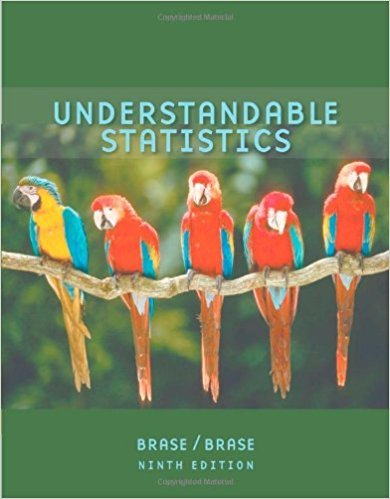×
×

# Solutions for Chapter 1.1: Getting Started## Full solutions for Understandable Statistics | 9th Edition

ISBN: 9780618949922Solutions for Chapter 1.1: Getting Started

Solutions for Chapter 1.1
4 5 0 416 Reviews
20
4
##### ISBN: 9780618949922

Since 13 problems in chapter 1.1: Getting Started have been answered, more than 34128 students have viewed full step-by-step solutions from this chapter. Understandable Statistics was written by and is associated to the ISBN: 9780618949922. This textbook survival guide was created for the textbook: Understandable Statistics, edition: 9. Chapter 1.1: Getting Started includes 13 full step-by-step solutions. This expansive textbook survival guide covers the following chapters and their solutions.

Key Statistics Terms and definitions covered in this textbook
• 2 k p - factorial experiment

A fractional factorial experiment with k factors tested in a 2 ? p fraction with all factors tested at only two levels (settings) each

• Arithmetic mean

The arithmetic mean of a set of numbers x1 , x2 ,…, xn is their sum divided by the number of observations, or ( / )1 1 n xi t n ? = . The arithmetic mean is usually denoted by x , and is often called the average

• Asymptotic relative eficiency (ARE)

Used to compare hypothesis tests. The ARE of one test relative to another is the limiting ratio of the sample sizes necessary to obtain identical error probabilities for the two procedures.

• Biased estimator

Unbiased estimator.

• Categorical data

Data consisting of counts or observations that can be classiied into categories. The categories may be descriptive.

• Central tendency

The tendency of data to cluster around some value. Central tendency is usually expressed by a measure of location such as the mean, median, or mode.

• Completely randomized design (or experiment)

A type of experimental design in which the treatments or design factors are assigned to the experimental units in a random manner. In designed experiments, a completely randomized design results from running all of the treatment combinations in random order.

• Conditional probability mass function

The probability mass function of the conditional probability distribution of a discrete random variable.

• Confounding

When a factorial experiment is run in blocks and the blocks are too small to contain a complete replicate of the experiment, one can run a fraction of the replicate in each block, but this results in losing information on some effects. These effects are linked with or confounded with the blocks. In general, when two factors are varied such that their individual effects cannot be determined separately, their effects are said to be confounded.

• Correction factor

A term used for the quantity ( / )( ) 1 1 2 n xi i n ? = that is subtracted from xi i n 2 ? =1 to give the corrected sum of squares deined as (/ ) ( ) 1 1 2 n xx i x i n ? = i ? . The correction factor can also be written as nx 2 .

• Correlation

In the most general usage, a measure of the interdependence among data. The concept may include more than two variables. The term is most commonly used in a narrow sense to express the relationship between quantitative variables or ranks.

• Correlation coeficient

A dimensionless measure of the linear association between two variables, usually lying in the interval from ?1 to +1, with zero indicating the absence of correlation (but not necessarily the independence of the two variables).

• Correlation matrix

A square matrix that contains the correlations among a set of random variables, say, XX X 1 2 k , ,…, . The main diagonal elements of the matrix are unity and the off-diagonal elements rij are the correlations between Xi and Xj .

• Deming’s 14 points.

A management philosophy promoted by W. Edwards Deming that emphasizes the importance of change and quality

• Empirical model

A model to relate a response to one or more regressors or factors that is developed from data obtained from the system.

• Erlang random variable

A continuous random variable that is the sum of a ixed number of independent, exponential random variables.

• Experiment

A series of tests in which changes are made to the system under study

• F-test

Any test of signiicance involving the F distribution. The most common F-tests are (1) testing hypotheses about the variances or standard deviations of two independent normal distributions, (2) testing hypotheses about treatment means or variance components in the analysis of variance, and (3) testing signiicance of regression or tests on subsets of parameters in a regression model.

• Gamma random variable

A random variable that generalizes an Erlang random variable to noninteger values of the parameter r

• Geometric random variable

A discrete random variable that is the number of Bernoulli trials until a success occurs.

×